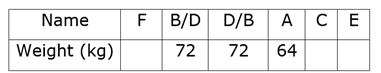# LIC Assistant Mains Reasoning Questions 2019 – (Day-3)

Dear Aspirants, Our IBPS Guide team is providing new pattern Reasoning Questions for LIC Assistant Mains 2019 so the aspirants can practice it on a daily basis. These questions are framed by our skilled experts after understanding your needs thoroughly. Aspirants can practice these new series questions daily to familiarize with the exact exam pattern and make your preparation effective.

Check here for LIC Assistant Mains Mock Test 2019

[WpProQuiz 7498]

Inequality

Directions (1-3): In each of the following questions relationship between different elements are given in the statement followed by three set of conclusions. Study the following information carefully and decide which of the following conclusion follows logically.

1) Statement:

F < B = M; W = G ≤ K; H < F = K; W > T = P;

Conclusion:

I) M > H

II) W < B

III) K ≥ P

a) Only II and III follows

b) All I, II and II follows

c) Only II follows

d) Only I and II follows

e) None follows

2) Statement:

R < Q ≥ M < H; M > T ≤ S = F; Q = L < W ≥ B

Conclusion:

I) R < W

II) H > F

III) L ≤ S

a) Only II and III follows

b) Only I follows

c) Only II follows

d) Only I and III follows

e) None follows

3) Statement:

D = F ≤ G < M; F < W = T; D > S ≤ K; G ≤ Z > H

Conclusion:

I) D ≤ T

II) M > S

III) H < M

a) Only II follows

b) Only I and II follows

c) Only III follows

d) Only I and III follows

e) None Follows

Puzzle

Directions (4-8): Study the following information carefully and answer the below questions.

Eight boxes marked B01 to B08 are kept one over in the form of stack. Each box contains different number of chocolates viz. 4, 6, 7, 8, 9, 12, 13 and 14. All the information are not necessary in same order. No two boxes marked with consecutive numbers are kept together.

Two boxes are kept between the box B06 and the box which contains 14 chocolates. The box B02 is kept at any place above the box B01, which contains perfect square number of chocolates. The box B03 is kept at a gap of three boxes below the box which contains 12 chocolate. The box which contains 12 chocolates are neither kept at top nor adjacent to the box which 4 chocolates. The box B08 doesn’t contains 14 chocolates. The box which contains 14 chocolates are kept at any place below the box B03. The box B04, which contains odd number of chocolates, is kept three places below the box which contains 6 chocolates. One box is kept between the box which contains 6 chocolates and the box B08. Number of boxes above the box which contains 13 chocolates is same as number of boxes below the box B08. Three boxes are kept between the box which contains 8 chocolates and the box B07. Number of boxes kept below the box which contains 8 chocolates is same as number of boxes above the box which contains 7 chocolates. At least two boxes are kept between the box which contains 9 chocolates and the box B05.

4) How many boxes are kept above the box which contains 7 chocolates?

a) One

b) Three

c) Two

d) More than three

e) None

5) Which of the following box contains 4 chocolates?

a) B07

b) B03

c) B06

d) B08

e) None of these

6) Which of the following box is kept just below the box which contains 9 chocolates?

a) B04

b) B02

c) B01

d) B09

e) None of these

7) Which of the following combination is odd among out of five combinations?

a) B02 – 9

b) B06 – 13

c) B01 – 8

d) B07 – 12

e) B08 – 7

8) What is sum of number of chocolates of the box B06 and the box B03?

a) 15

b) 21

c) 23

d) 17

e) None of these

Order and Ranking

Directions (9-10): Study the following information carefully and answer the below questions.

Weight of six persons namely – A, B, C, D, E and F. Weight of C is more than E but not as much as F, D. Weight of B is more than A and E but not as much as F. Weight of F is greater than D. Weight of D is 72kg and weight of the one whose weight is fourth highest is 64kg. Weight of A is more than C but not as much as D.

9) What is possible weight of B, if weight of heaviest person is 92kg?

a) 76kg

b) 84kg

c) 54kg

d) 68kg

e) Can’t be determined.

10) Weight of who among the following person is 64kg?

a) C

b) A

c) E

d) F

e) Can’t be determined.

Given Statement: F < B = M; W = G ≤ K; H < F = K; W > T = P;

From I: M > H    (M = B > F > H) àTrue

From II: W<B     (B > F = K ≥ G = W) àTrue

From III: K ≥ P   (K ≥ G = W > T ≥ P) à False

Hence, option D is correct choice.

Given Statement: R < Q ≥ M < H; M > T ≤ S = F; Q = L < W ≥ B

From I: R < W    (R < Q = L < W) à True

From II: H > F    (H > M > T ≤ S = F) àFalse

From III: L ≤ S   (L = Q ≥ M > T ≤ S) à False

Hence, option B is correct choice.

Given Statement: D = F ≤ G < M; F < W = T; D > S ≤ K; G ≤ Z > H

From I: D ≤ T     (D = F < W = T) –> False

From II: M > S   (M > G ≥ F = D > S) –> True

From III: H < M  (H < Z ≥ G < M) –> False

Hence, option A is correct choice.

Directions (4-8):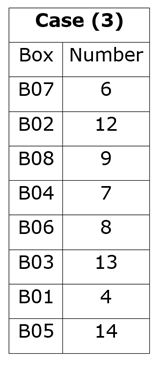We have:

• The box B03 is kept at a gap of three boxes below the box which contains 12 chocolate.
• The box which contains 12 chocolates are neither kept at top nor adjacent to the box which 4 chocolates.
• Two boxes are kept between the box B06 and the box which contains 14 chocolates.
• The box which contains 14 chocolates are kept at any place below the box B03.

That means, we have two possible place for the box which contains 14 chocolates, in case (1) The box B03 is kept second from bottom and the box which contains 14 chocolates are kept at bottom, in case (2) the box B03 is kept third from bottom and the box which contains 14 chocolates are kept second from bottom, in case (3) the box B03 is kept third from bottom and the box which contains 14 chocolates are kept at bottom.

Based on above given information we have: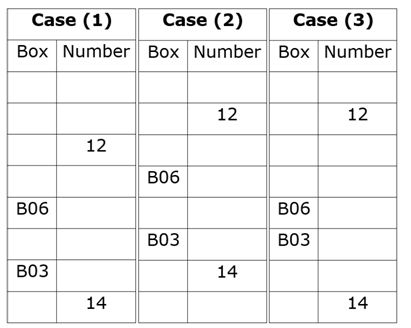Again, we have:

• The box B04, which contains odd number of chocolates, is kept three places below the box which contains 6 chocolates.
• One box is kept between the box which contains 6 chocolates and the box B08.
• The box B08 doesn’t contains 14 chocolates.
• Number of boxes above the box which contains 13 chocolates is same as number of boxes below the box B08.

Since, no two boxes marked with consecutive number are kept together.

That means, in case (1) & case (3) the box B04 is kept just above the box B06, in case (2) the box B04 is kept at bottom.

• Three boxes are kept between the box which contains 8 chocolates and the box B07.
• Number of boxes kept below the box which contains 8 chocolates is same as number of boxes above the box which contains 7 chocolates.

That means, in case (1) & case (3) the box B07 is kept at top, case (2) is not valid.

Based on above given information we have: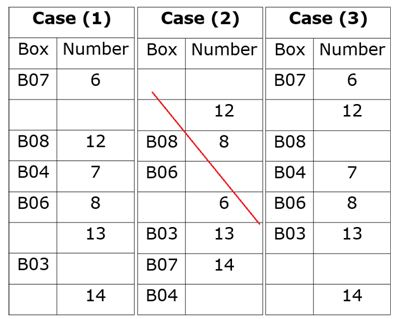Case (2) is not valid as number of boxes kept below the box which contains 8 chocolates is same as number of boxes above the box which contains 7 chocolates.

Again, we have:

• The box B02 is kept at any place above the box B01, which contains perfect square number of chocolates.

Since, no two box marked with consecutive number are kept together.

That means, in case (3) the box B01 is kept just below the box B03, case (1) is not valid.

• At least two boxes are kept between the box which contains 9 chocolates and the box B05.

That means, the box B05 is kept at bottom and the box B08 contains 9 chocolates.

Based on above given information we have final arrangement as follows: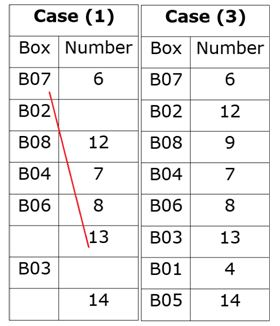Case (1) is not valid as the box B01 contains perfect square number of chocolates.

Clearly, only three boxes are kept above the box which contains 7 chocolates.

Hence, option B is correct choice.

Clearly, only three boxes are kept above the box which contains 7 chocolates.

Hence, option B is correct choice.

Clearly, the box B01 contains 4 chocolates.

Hence, option E is correct choice.

Clearly, the box B04 is kept just below the box which contains 9 chocolates.

Hence, option A is correct choice.

Clearly, 2nd element is kept just below the 1st article.

Hence, option C is odd among all five.

Clearly, required sum = (13 + 8) = 21

Hence, option B is correct choice.

Clearly, exact position of B is not known.

Hence, option E is correct choice.

Clearly, weight of A is 64kg.

Hence, option B is correct choice.

Common Explanation:

We have:

• Weight of B is more than A and E but not as much as F.

F > B > A, E

• Weight of C is more than E but not as much as F, D.

F, D > C > E

• Weight of F is greater than D.
• Weight of A is more than C but not as much as D.

Thus, after combining above statements we have:

F > B, D > A > C > E

• Weight of D is 72kg and weight of the one whose weight is fourth highest is 64kg.

That means, weight of A must be 64kg.

Based on above given information we have final arrangement as follows: